# Liouville equation

(diff) ← Older revision | Latest revision (diff) | Newer revision → (diff)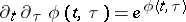The Liouville equation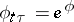(a1)

is a non-linear partial differential equation (cf. Differential equation, partial) that can be linearized and subsequently solved. Namely, it can be transformed into the linear wave equation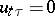(a2)

by any of the following two differential substitutions (see [a1], formulas (4) and (2)):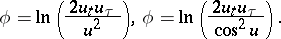(a3)

In other words, the formulas (a3) provide the general solution to the Liouville equation, in terms of the well-known general solution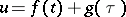of the wave equation (a2).

The Liouville equation appears also in Lie's classification [a2] of second-order differential equations of the form(a4)

For the complete classification, see [a4].

The Liouville equation (a1) is invariant under the infinite group of point transformations(a5)

with arbitrary invertible differentiable functions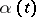and. The infinitesimal generator of this group is:where,are arbitrary functions and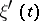,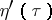are their first derivatives. It is shown in [a2] that the equation (a4), and in particular the Liouville equation, does not admit non-trivial (i.e. non-point) Lie tangent transformations.

In addition to the transformations (a3), it is known (see, e.g., [a3]) that the Liouville equation is related with the wave equation (a2) by the following Bäcklund transformation:By letting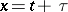,in (a1), (a2) and (a3), where, one can transform the elliptic Liouville equation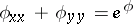into the Laplace equation.

How to Cite This Entry:
Liouville equation. Encyclopedia of Mathematics. URL: http://encyclopediaofmath.org/index.php?title=Liouville_equation&oldid=18774
This article was adapted from an original article by N.H. Ibragimov (originator), which appeared in Encyclopedia of Mathematics - ISBN 1402006098. See original article